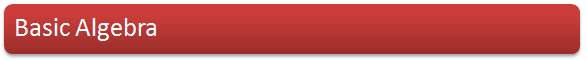Collecting Like Terms, Factorisation, Multiplication & Fractions
Simultaneous Equations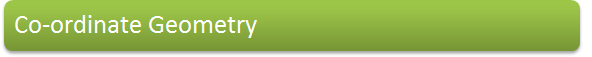The distance between two points
The mid-point of a line joining two points
The equation of straight line
The intersection of two lines
Curves
The circle
The intersection of a line and a curve & two curves
Key Points of this Module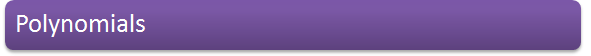Operations with polynomials
Polynomial curves
Using transformations to sketch the curves of functions
Binomial expansions
Key Points of this Module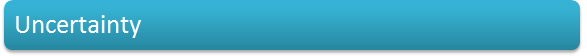Errors and inequalities
The algebra of inequalities
Key Points of this Module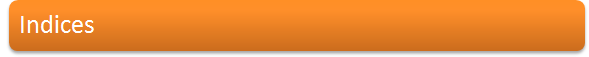Working with square roots
Negative and fractional indices
Key Points of this Module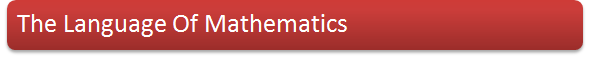Types of numbers
Writing mathematics
The converse of a theorem
Proof
Key Points of this ModuleJanuary 2001 (OCR MEI)
January 2005 (OCR MEI)Revision Sheet
Forumla You Need To Know